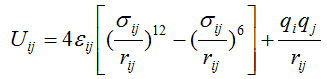# what's the value of energy-conversion

Dear Mr Tiwari,

Several days ago, I asked a question that I was confused by the formula for potential in some MD paper based on LAMMPS. The interaction between atomic sites are expressed asFor the Coulombic potential, It seems that both of the energy-conversion constant C and the dielectric constant equal 1.

Thanks for your reply and I have done a test run as you recommend: two atoms with the charge of 1 C are put in a box, and the distance is set to 1m, in addition, I take the “coul/cut” potential, and the “units” is set to SI. Coulomb potential is export by the thermos_style command, and the result is 8.9876109. This value is equal to (1/4piepsilon). Therefore, I think 1/4piepsilon cannot be omitted in the Potential equation. However, I have found some published paper that omit 1/4pi*epsilon. Can you give me some clear explanation.

Thanks!

Zhang Chao

Hi Zhang,

1/(4piepsilon0) is surely part of Coulomb potential. I tested it many time, even with your chosen form of potential. I am again repeating the steps which may help you somewhat, else let me know, i will share the example input after digging my example repository.

1. Take # Force field with SI units

pair_style lj/cut/coul/cut {cutf} pair_coeff * * {elj} \${sig}

1. Take as many as particles anywhere in box (just remember box size and take cutoff smaller than box size for the check purpose)

2. use command pair_write to write potential energy between any two particles at range of distances. Plot it.
The text should be something like
pair_write 1 2 2000 r {icf} {ocf} pot12.dat lj/coul {Q1} {Q2}

3. Analytically plot the same potential in that spacial range on the top of it. Both must match.

This clearly will reflect that 1/4piepsilon0 is part of potential code.
I remember Axel gave one more hint to look into some file for the same thread.

Hope it works.

Sanat